Sound Waves and Music - Lesson 5 - Physics of Musical Instruments

# Open-End Air Columns

Many musical instruments consist of an air column enclosed inside of a hollow metal tube. Though the metal tube may be more than a meter in length, it is often curved upon itself one or more times in order to conserve space. If the end of the tube is uncovered such that the air at the end of the tube can freely vibrate when the sound wave reaches it, then the end is referred to as an open end. If both ends of the tube are uncovered or open, the musical instrument is said to contain an open-end air column. A variety of instruments operate on the basis of open-end air columns; examples include the flute and the recorder. Even some organ pipes serve as open-end air columns.

### Standing Wave Patterns for the Harmonics

As has already been mentioned, a musical instrument has a set of natural frequencies at which it vibrates at when a disturbance is introduced into it. These natural frequencies are known as the harmonics of the instrument; each harmonic is associated with a standing wave pattern. In Lesson 4 of Unit 10, a standing wave pattern was defined as a vibrational pattern created within a medium when the vibrational frequency of the source causes reflected waves from one end of the medium to interfere with incident waves from the source in such a manner that specific points along the medium appear to be standing still. In the case of stringed instruments (discussed earlier), standing wave patterns were drawn to depict the amount of movement of the string at various locations along its length. Such patterns show nodes - points of no displacement or movement - at the two fixed ends of the string. In the case of air columns, a closed end in a column of air is analogous to the fixed end on a vibrating string. That is, at the closed end of an air column, air is not free to undergo movement and thus is forced into assuming the nodal positions of the standing wave pattern. Conversely, air is free to undergo its back-and-forth longitudinal motion at the open end of an air column; and as such, the standing wave patterns will depict antinodes at the open ends of air columns.

So the basis for drawing the standing wave patterns for air columns is that vibrational antinodes will be present at any open end and vibrational nodes will be present at any closed end. If this principle is applied to open-end air columns, then the pattern for the fundamental frequency (the lowest frequency and longest wavelength pattern) will have antinodes at the two open ends and a single node in between. For this reason, the standing wave pattern for the fundamental frequency (or first harmonic) for an open-end air column looks like the diagram below.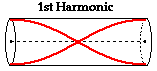The distance between antinodes on a standing wave pattern is equivalent to one-half of a wavelength. A careful analysis of the diagram above shows that adjacent antinodes are positioned at the two ends of the air column. Thus, the length of the air column is equal to one-half of the wavelength for the first harmonic.

The standing wave pattern for the second harmonic of an open-end air column could be produced if another antinode and node was added to the pattern. This would result in a total of three antinodes and two nodes. This pattern is shown in the diagram below. Observe in the pattern that there is one full wave in the length of the air column. One full wave is twice the number of waves that were present in the first harmonic. For this reason, the frequency of the second harmonic is two times the frequency of the first harmonic.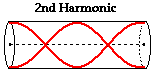And finally, the standing wave pattern for the third harmonic of an open-end air column could be produced if still another antinode and node were added to the pattern. This would result in a total of four antinodes and three nodes. This pattern is shown in the diagram below. Observe in the pattern that there are one and one-half waves present in the length of the air column. One and one-half waves is three times the number of waves that were present in the first harmonic. For this reason, the frequency of the third harmonic is three times the frequency of the first harmonic.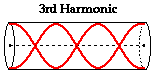### Summary of Length-Wavelength Relationships

The process of adding another antinode and node to each consecutive harmonic in order to determine the pattern and the resulting length-wavelength relationship could be continued. If doing so, it is important to keep antinodes on the open ends of the air column and to maintain an alternating pattern of nodes and antinodes. When finished, the results should be consistent with the information in the table below.

The relationships between the standing wave pattern for a given harmonic and the length-wavelength relationships for open end air columns are summarized in the table below.

 Harm. # # of Waves in Air Column # of Nodes # of Antinodes Length- Wavelength Relationship 1 1/2 1 2 Wavelength = (2/1)*L 2 1 or 2/2 2 3 Wavelength = (2/2)*L 3 3/2 3 4 Wavelength = (2/3)*L 4 2 or 4/2 4 5 Wavelength = (2/4)*L 5 5/2 5 6 Wavelength = (2/5)*L

### Problem-Solving Scheme

Now the aim of the above discussion is to internalize the mathematical relationships for open-end air columns in order to perform calculations predicting the length of air column required to produce a given natural frequency. And conversely, calculations can be performed to predict the natural frequencies produced by a known length of air column. Each of these calculations requires knowledge of the speed of a wave in air (which is approximately 340 m/s at room temperatures). The graphic below depicts the relationships between the key variables in such calculations. These relationships will be used to assist in the solution to problems involving standing waves in musical instruments.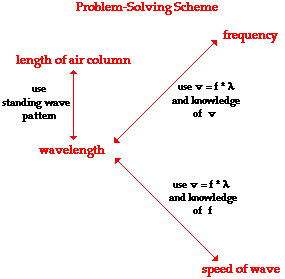To demonstrate the use of the above problem-solving scheme, consider the following example problem and its detailed solution.

### Example Problem #1

The speed of sound waves in air is found to be 340 m/s. Determine the fundamental frequency (1st harmonic) of an open-end air column that has a length of 67.5 cm.

The solution to the problem begins by first identifying known information, listing the desired quantity, and constructing a diagram of the situation.

 Given: v = 340 m/s L = 67.5 cm = 0.675 m Find: f1 = ?? Diagram: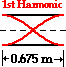The problem statement asks us to determine the frequency (f) value. From the graphic above, the only means of finding the frequency is to use the wave equation (speed = frequency • wavelength) and knowledge of the speed and wavelength. The speed is given, but wavelength is not known. If the wavelength could be found then the frequency could be easily calculated. In this problem (and any problem), knowledge of the length and the harmonic number allows one to determine the wavelength of the wave. For the first harmonic, the wavelength is twice the length. This relationship is derived from the diagram of the standing wave pattern (see table above). The relationship, which works only for the first harmonic of an open-end air column, is used to calculate the wavelength for this standing wave.

Wavelength = 2 • Length

Wavelength = 2 • 0.675 m

Wavelength = 1.35 m

Now that wavelength is known, it can be combined with the given value of the speed to calculate the frequency of the first harmonic for this open-end air column. This calculation is shown below.

speed = frequency • wavelength

frequency = speed / wavelength

frequency = (340 m/s) / (1.35 m)

frequency = 252 Hz

Most problems can be solved in a similar manner. It is always wise to take the extra time needed to set the problem up. Take the time to write down the given information and the requested information, and to draw a meaningful diagram.

Seldom in physics are two problems identical. The tendency to treat every problem the same way is perhaps one of the quickest paths to failure. It is much better to combine good problem-solving skills (part of which involves the discipline to set the problem up) with a solid grasp of the relationships among variables. Avoid the tendency to memorize approaches to different types of problems.

To further your understanding of these relationships and the use of the above problem-solving scheme, consider the following example problem and its detailed solution.

### Example Problem #2

Determine the length of an open-end air column required to produce a fundamental frequency (1st harmonic) of 480 Hz. The speed of waves in air is known to be 340 m/s.

The solution to the problem begins by first identifying known information, listing the desired quantity, and constructing a diagram of the situation.

 Given: v = 340 m/s f1 = 480 Hz Find: L = ?? Diagram: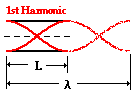The problem statement asks us to determine the length of the air column. When inspecting the problem-solving scheme described above, one will notice that the only means of finding the length of the air column is from knowledge of the wavelength. But the wavelength is not known. However, the frequency and speed are given, so one can use the wave equation (speed = frequency • wavelength) and knowledge of the speed and frequency to determine the wavelength. This calculation is shown below.

speed = frequency • wavelength

wavelength = speed / frequency

wavelength = (340 m/s) / (480 Hz)

wavelength = 0.708 m

Now that the wavelength is found, the length of the air column can be calculated. For the first harmonic, the length is one-half the wavelength. This relationship is derived from the diagram of the standing wave pattern (see table above). The relationship may also be evident to you by looking at the standing wave diagram drawn above. This relationship between wavelength and length, which works only for the first harmonic of an open-end air column, is used to calculate the wavelength for this standing wave.

Length = (1/2) • Wavelength

Length = (1/2) • Wavelength

Length = 0.354 m

If you have successfully managed the above two problems, take a try at the following practice problems. As you proceed, be sure to be mindful of the numerical relationships involved in such problems. And if necessary, refer to the graphic above.

### We Would Like to Suggest ...Why just read about it and when you could be interacting with it? Interact - that's exactly what you do when you use one of The Physics Classroom's Interactives. We would like to suggest that you combine the reading of this page with the use of our Standing Wave Patterns Interactive. You can find it in the Physics Interactives section of our website. The Standing Wave Patterns Interactive provides the learner an environment for exploring the formation of standing waves, standing wave patterns, and mathematical relationships for standing wave patterns.

### Check Your Understanding

1. Stan Dinghwaives is playing his open-end pipe. The frequency of the second harmonic is 880 Hz (a pitch of A5). The speed of sound through the pipe is 350 m/sec. Find the frequency of the first harmonic and the length of the pipe.

2. On a cold frigid day, Matthew blows on a toy flute, causing resonating waves in an open-end air column. The speed of sound through the air column is 336 m/sec. The length of the air column is 30.0 cm. Calculate the frequency of the first, second, and third harmonics.

3. A flute is played with a first harmonic of 196 Hz (a pitch of G3). The length of the air column is 89.2 cm (quite a long flute). Find the speed of the wave resonating in the flute.

Next Section: